# Linear Equations in Two Variables Class 10 NCERT Solutions

## Class 10 Maths Chapter 3 NCERT Solutions

### NCERT Solutions Class 10 Maths Chapter 3 PDF

Students learn to demonstrate real-life situations mathematically in terms of a pair of linear equations in class 10 maths chapter 3. By practising the NCERT solutions for class 10 maths chapter 3 Pair Of Linear Equations In Two Variables provided below, students clearly understand the four methods used to solve a pair of linear equations. The following are the four methods:

• Substitution Method
• Graphical methods
• Cross- Multiplication Method
• Elimination Method

To learn everything there is to know about to solve a pair of linear equations in two variables, make the best use of the solutions provided below and attain great marks in the class 10 math exam.

### Understanding Pair of linear equations in two variables:

Consider the following linear equations in two variables.
3x+2y=5
x-2y=3
An equation which can be written in the form ax+by+c=0 , where a,b,c are real numbers and a and b are both non zero, is called a linear equation in two variables x and y. The solution of this type of equation is a pair of values, one for x and other for y.
Consider the equation 3x+2y=5. Substitute x=1 and y=1 on LHS.
Then LHS = (3×1)+(2×1)=5 which is equal to RHS of the equation. So, x=1 and y=1 is a solution of this equation 3x+2y=5.
Now put x=2 and y=3 in the equation 3x+2y=5
Then LHS = (3×2)+(2×3)=12 which is not equal to RHS. So x=2 and y=3 is not a solution of the equation.
Geometrically, it means the point (1,1) lies on the line representing the equation 3x+2y=5 and the point (2,3) does not lie on it. Therefore, every solution of the equation is a point which lies on the line which represents it.
Exercise 3.3
Solve the following pair of linear equations by substitution method.
x+y = 14 and x-y =4
x+y = 14 …(1)
x-y =4 …(2)
From equation (1) we can write x=14-y.
Substitute x in equation (2)
14-y-y=4
14-2y=4
-2y=-10
y=(-10)/(-2)
y=5
Substitute y=5 in equation (2)
x-5=4
x=4+5
x=9
So, x=9 and y=5

If two linear equations have the two same variables, they are called a pair of linear equations in two variables. Following is the most general form of linear equations:

a1 x + b1 y + c1 =0

a2 x + b2 y + c2 = 0

Here, al, a2, b1, b2, cl and c2 are real numbers such that;

a12 + b12 ≠ 0, a22 + b22 ≠ 0

A pair of linear equations can be represented and solved by the following methods:

1. Graphical method
2. Algebraic method

Exercise 3.1 : Solutions of Questions on Page Number 44

Q1 :

Aftab tells his daughter, “Seven years ago, I was seven times as old as you were then. Also, three years from now, I shall be three times as old as you will be.” (Isn’t this interesting?) Represent this situation algebraically and graphically.

Answer : Let the present age of Aftab be x.

And, present age of his daughter = y

Seven years ago,

Age of Aftab = x – 7

Age of his daughter = y – 7

According to the question,

(x-7)= 7(y-7)

x- 7 =7y-49

x — 7y = —42 (I)

Three years hence,

Age of Aftab = x + 3

Age of his daughter = y + 3

According to the question,

(x+3)=3(y+3)

x+3 =3y+9

x-3y=6 (2)

Therefore, the algebraic representation is

x -7y = -42

x -3y = 6

For x – 7y = -42

x=-42+7y

The solution table is

 x -7 0 7 y 5 6 7

For x -3y = 6

x = 6+3y

The solution table is

 x 6 3 0 y 0 -1 -2The coach of a cricket team buys 3 bats and 6 balls for Rs 3900. Later, she buys another bat and 3 more balls

of the same kind for Rs 1300. Represent this situation algebraically and geometrically.

Let the cost of a bat be Rs x.

And, cost of a ball = Rs y

According to the question, the algebraic representation is

[Math Processing Error]

For 3x + 6y = 3900

X= (3900-6y)/3

The solution table is

 x 300 100 -100 y 500 600 700

For x + 3y = 1300,

X = 1300-2y

The solution table is

 x 400 700 1000 y 300 200 100

The graphical representation for the first line is as follows.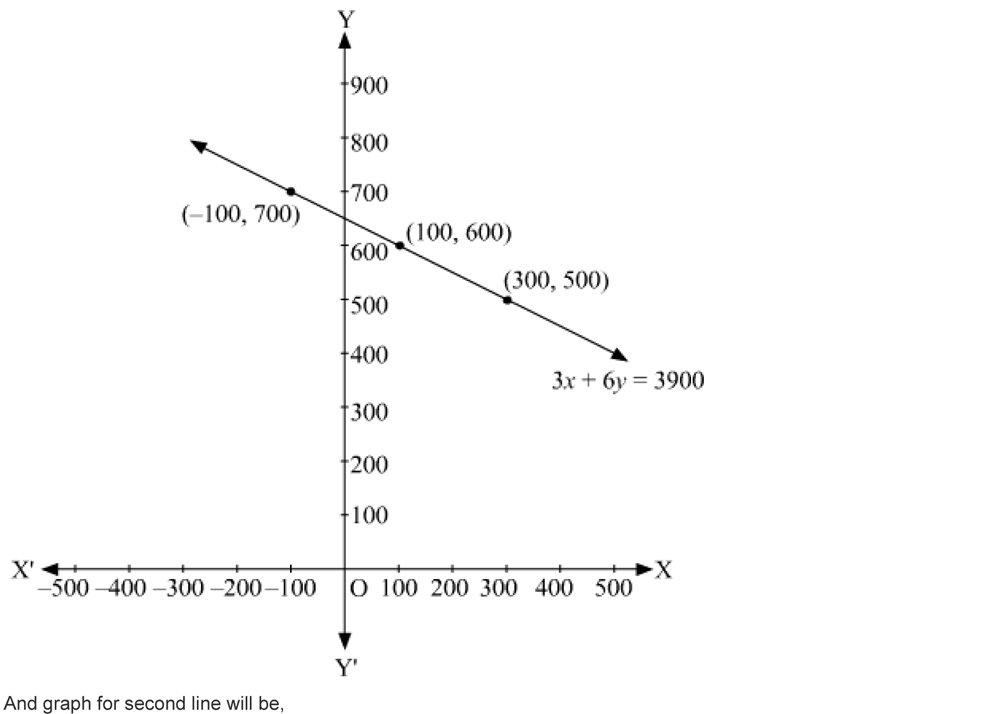The cost of 2 kg of apples and 1 kg of grapes on a day was found to be Rs 160. After a month, the cost of 4 kg of apples and 2 kg of grapes is Rs 300. Represent the situation algebraically and geometrically.

Let the cost of 1 kg of apples be Rs x.

And, cost of 1 kg of grapes = Rs y

According to the question,

the algebraic representation is 2x + y =160

4x + 2y =300

For 2x + y = 160,

y= 160-2x

The solution table is

 x 50 60 70 y 60 40 20

for 4x + 2y = 300,

y= (300-4x)/2

The solution table is

 x 70 80 75 y 10 -10 0

The graphical representation is as follows:Exercise 3.2 : Solutions of Questions on Page Number : 49
Q1:

Form the pair of linear equations in the following problems, and find their solutions graphically.

(i) 10 students of Class X took part in a Mathematics quiz. If the number of girls is 4 more than the number of
boys, find the number of boys and girls who took part in the quiz.

(ii) 5 pencils and 7 pens together cost Rs 50, whereas 7 pencils and 5 pens together cost Rs 46. Find the cost
of one pencil and that of one pen.

(i) Let the number of girls be x and the number of boys be y.

According to the questions, the algerbraic representation is

x + y = 10

x – y = 4

for x + y = 10

x=10-y

 x 5 4 6 y 5 6 4

For x-y = 4

x = 4+y

 x 5 4 3 y 1 0 -1

Hence, the graphics representation is as follows.

From the figure, it can be observed that these lines intersect each other at point (7, 3).
Therefore, the number of girls and boys in the class are 7 and 3 respectively.
(ii) Let the cost of 1 pencil be Rs x and the cost of 1 pen be Rs y.
According to the question, the algebraic representation is
5x + 7y = 50

7x + 5y = 46

For 5x + 7y = 50,

x=(50-7y)/5

 x 3 10 -4 y 5 0 10

7x + 5y = 46

x=(46-5y)/7

 x 8 3 -2 y -2 5 12

Hence, the graphic representation is as follows,From the figure, it can be observed that these lines intersect each point (3,5)

Therefore, the cost of a pencil and a pen are Rs.3 and Rs.5 respectively

Q2:

On comparing the ratios, a1/a2, b1/b2, and c1/c2 find out whether the lines representing the following pairs of linear equation at a point, are parrallel or coincident.

(i) 5x – 4y + 8 = 0 (ii) 9x + 3y + 12 = 0 (iii) 6x – 3y + 10 = 0

7x + 6y – 9 = 0 18x + 6y +24 =0 2 x – y + 9 = 0

(i) 5x- 4y+8=0

7x + 6y-9=0

Comparing these equations with a1x + b1y + c1 = 0

And a2x + b2 y + c2 =0 , we obtain

a1=5, b2=-4, c1=8

a2=7, b2 = 6, c2 = -9

a1/a2 = 5/7

b1 / b2 = -4/6 = -2/3

Since a1/a2 ≠ b1 / b2

Hence, the lines representing the given pair of equations have a unique solution and the pair of lines intersects at

exactly one point.

(ii) 9x + 3y+12=0

18x + 6y + 24=0

Comparing these equations with a1x + b1y + c1 = 0

And a2x + b2 y + c2 =0 we obtain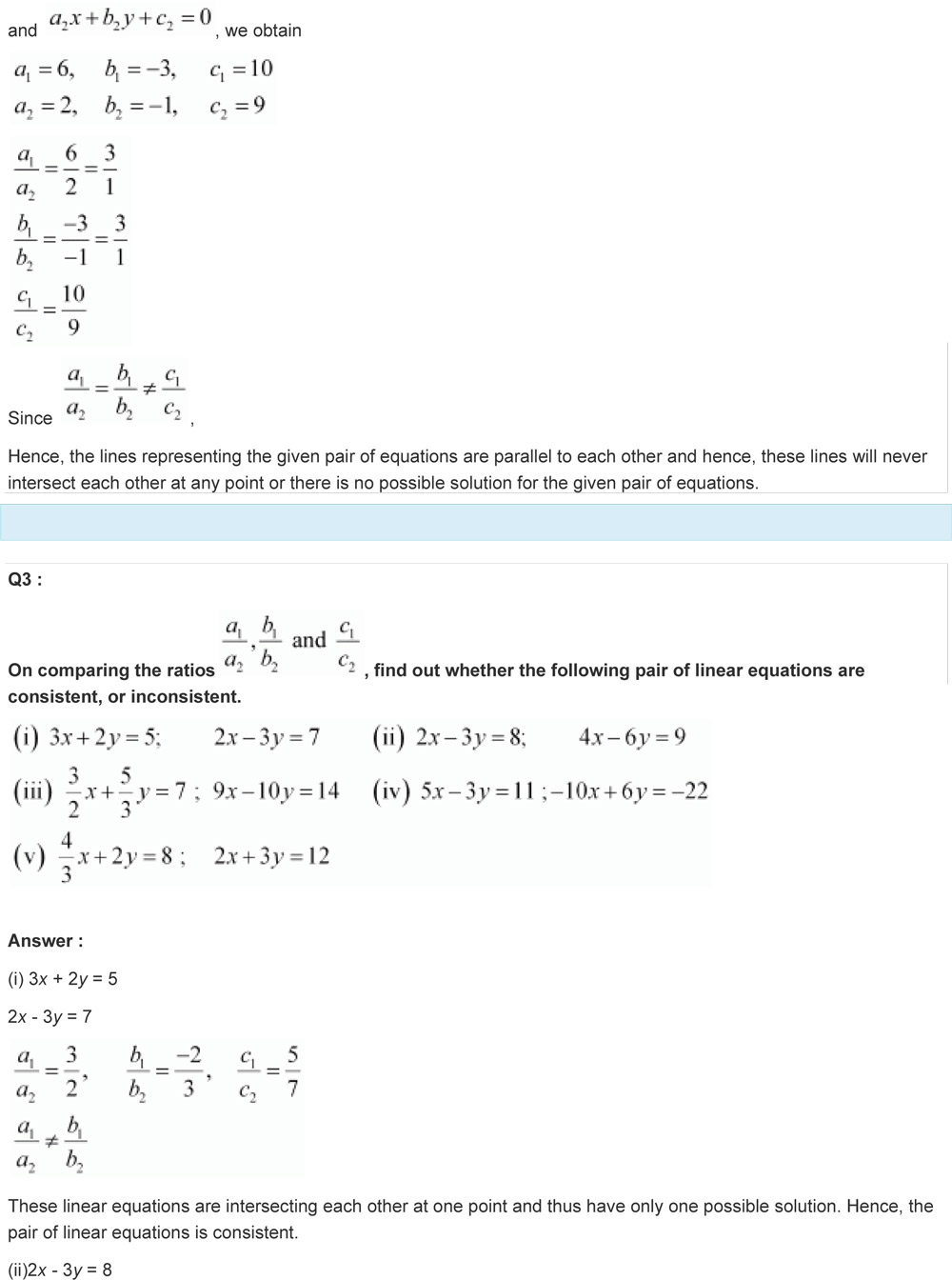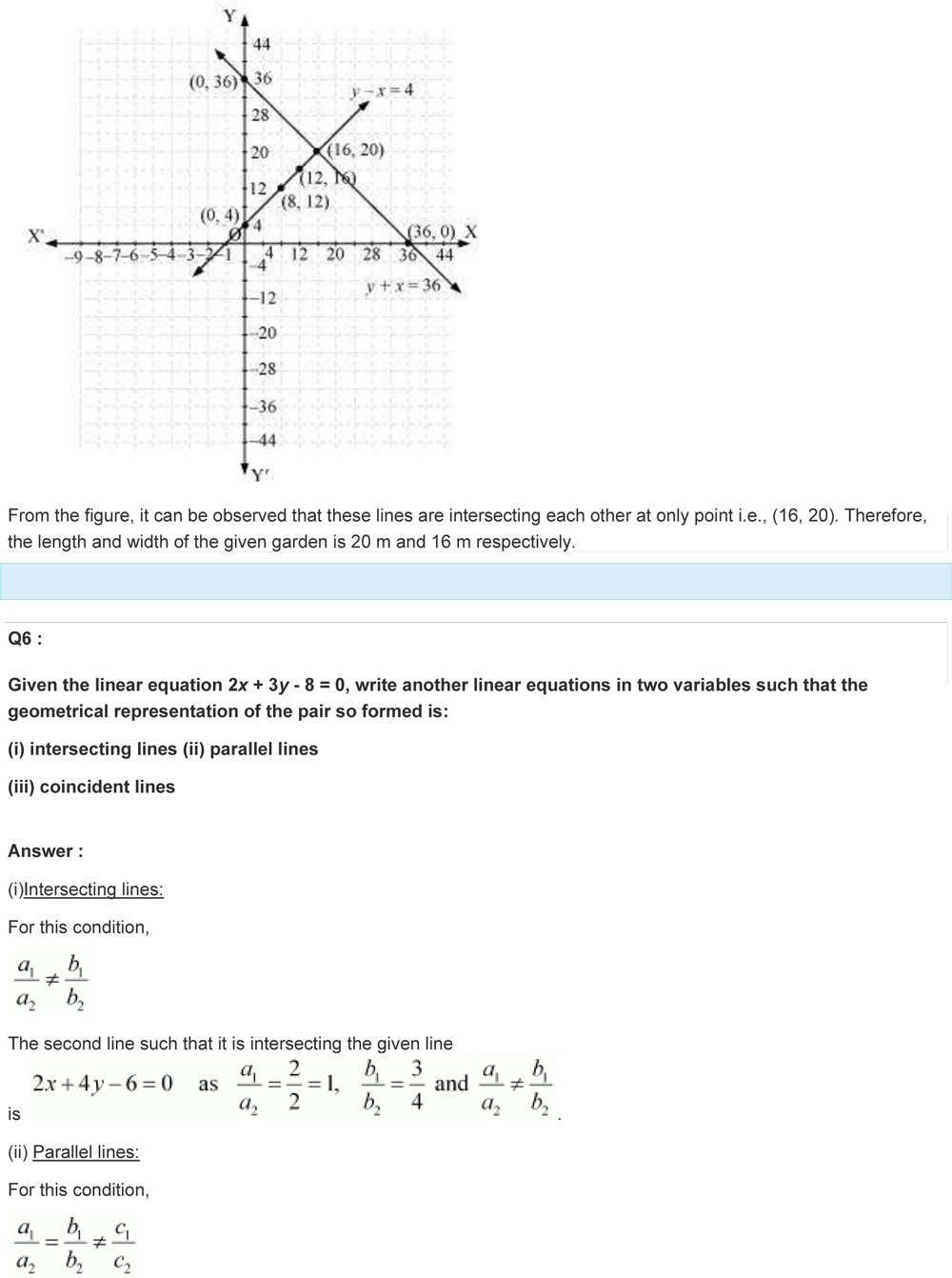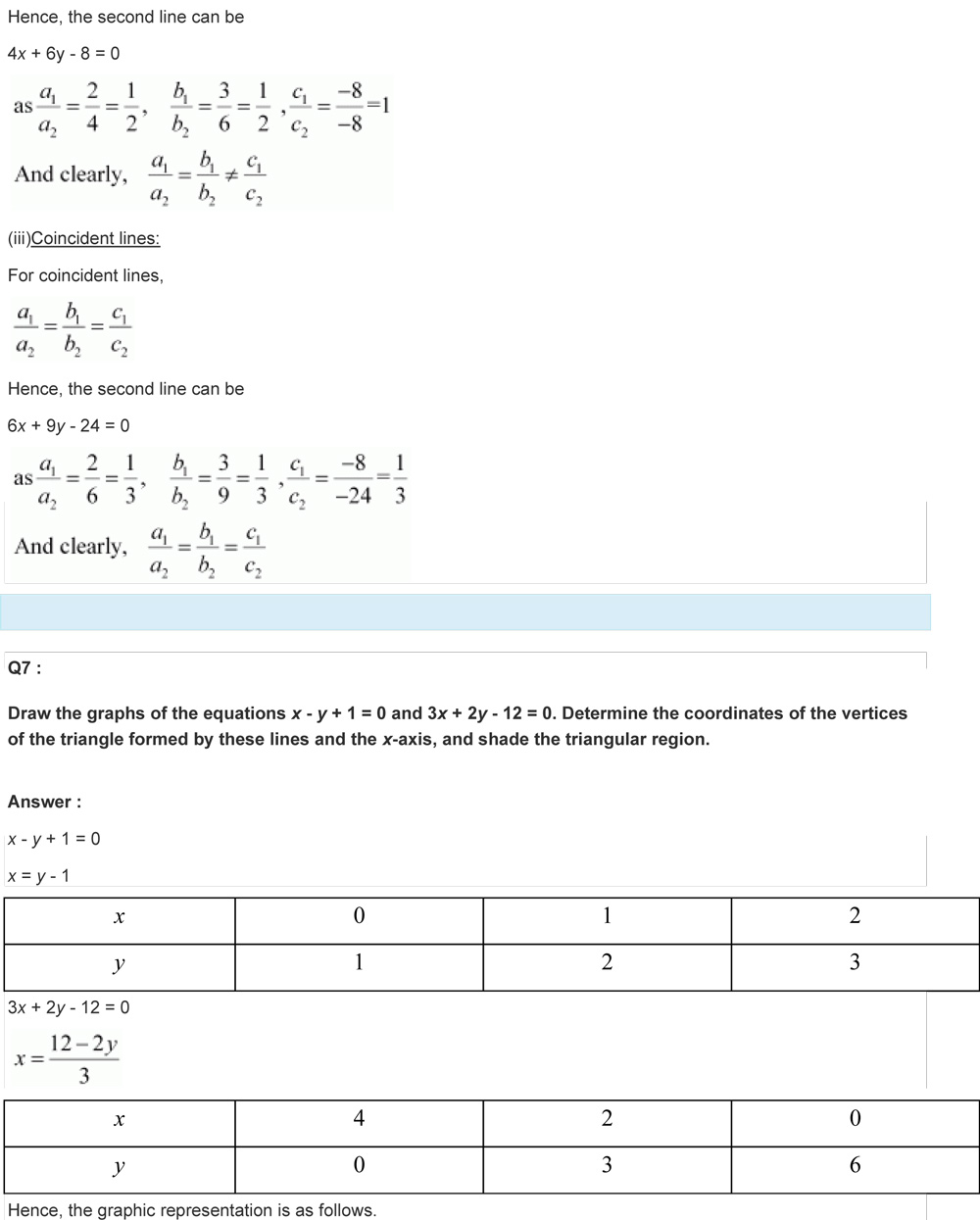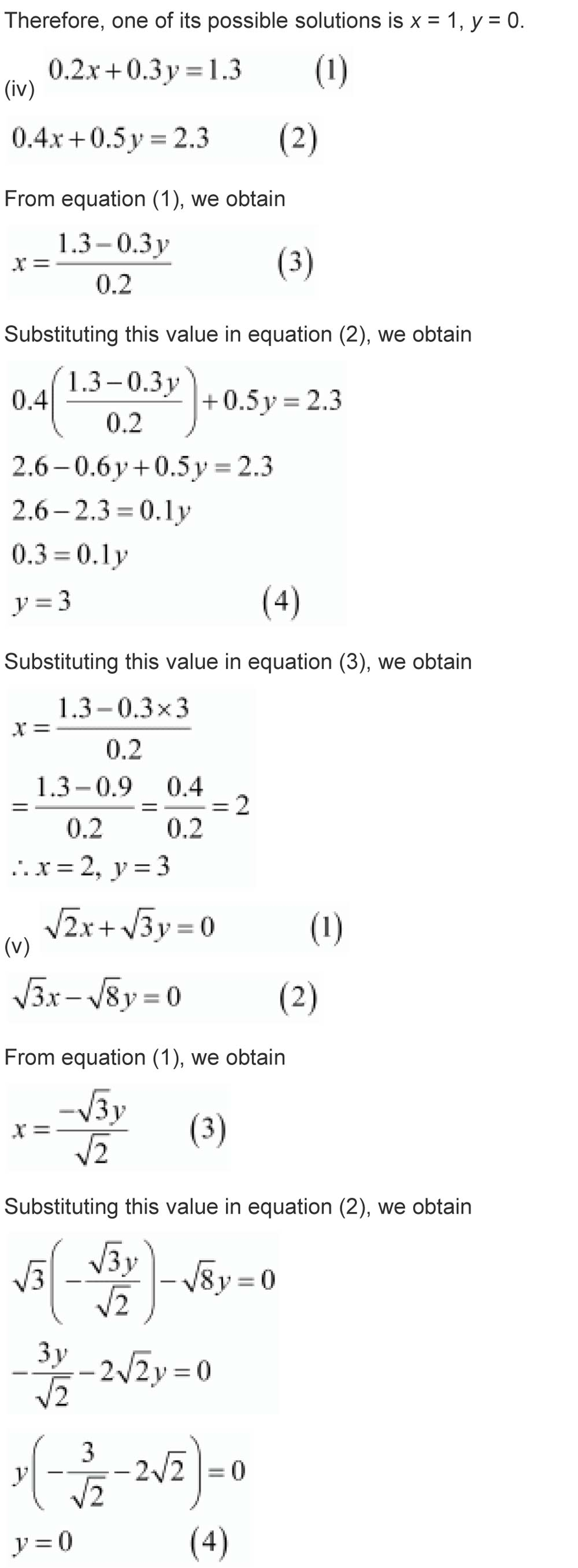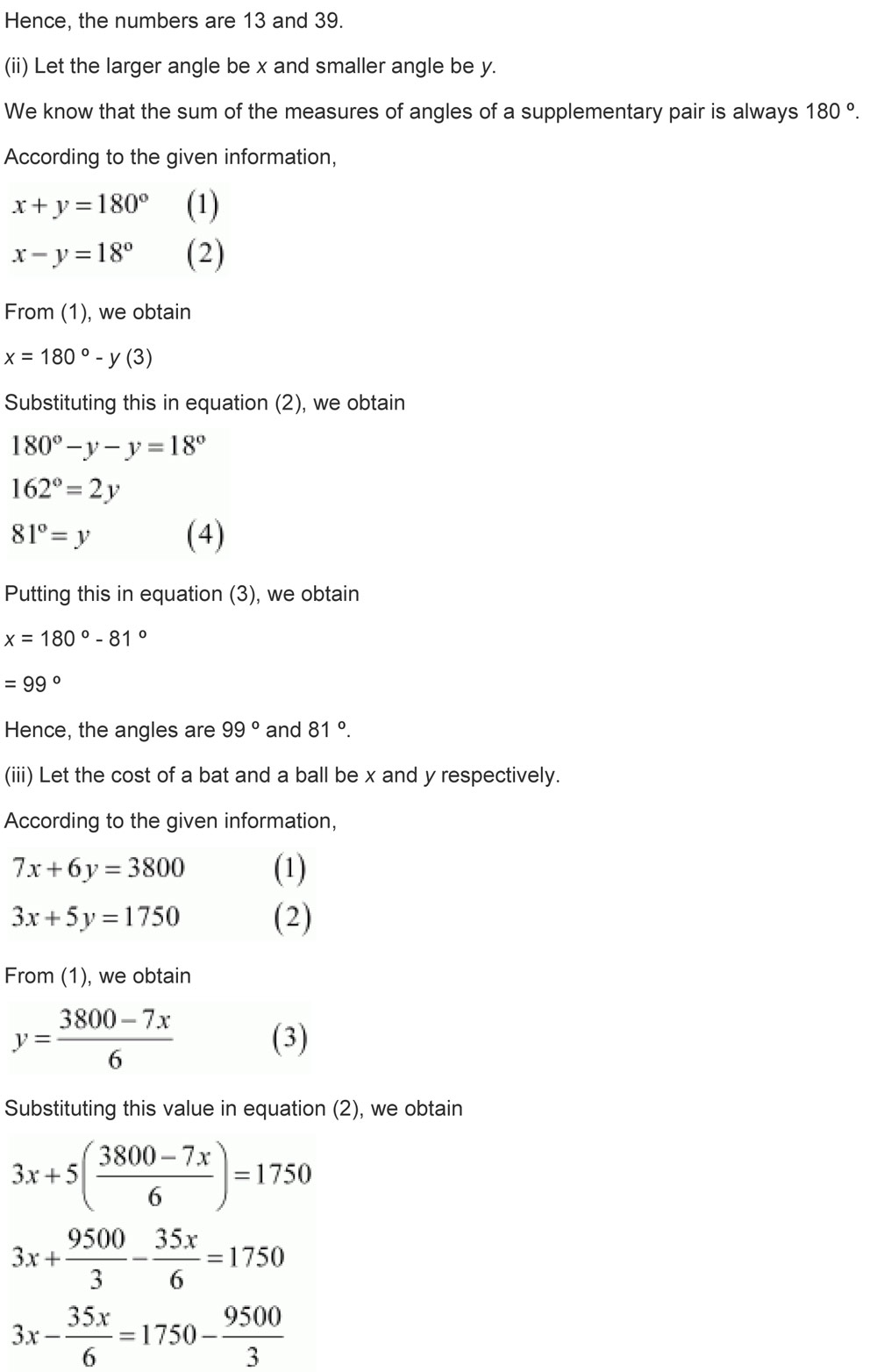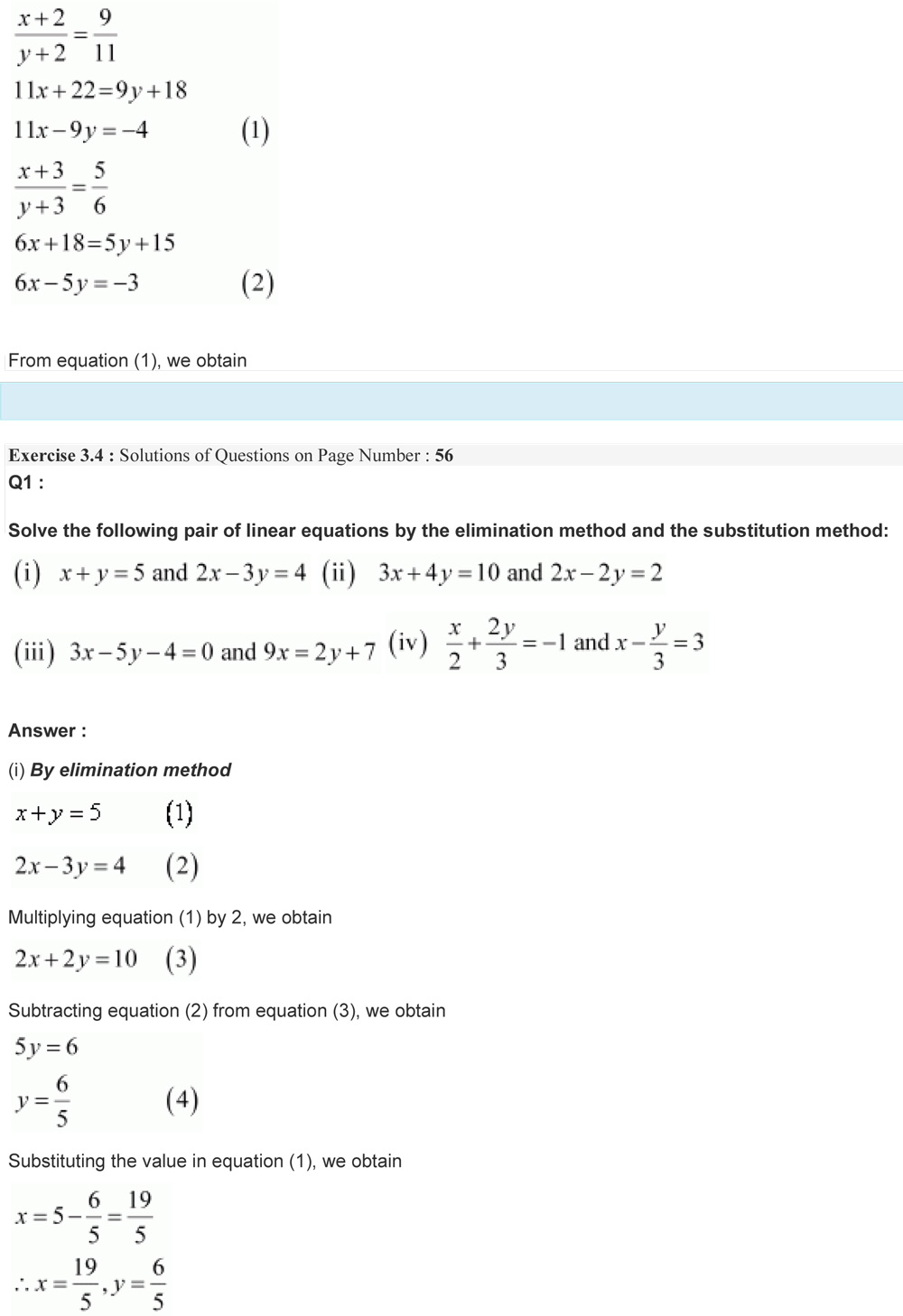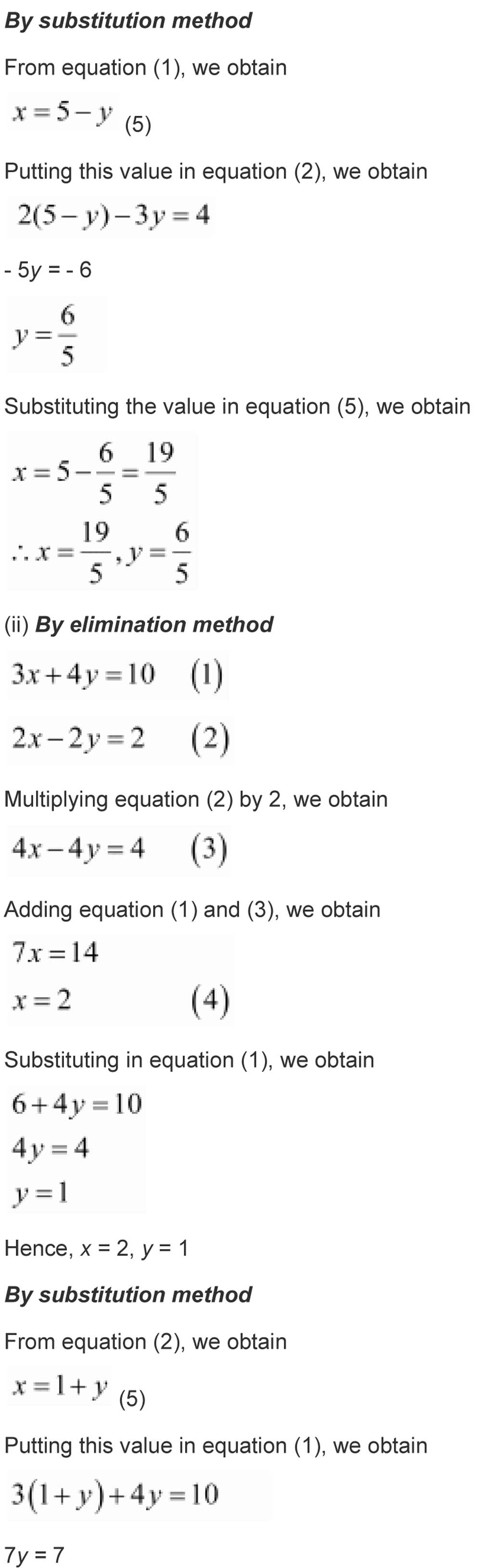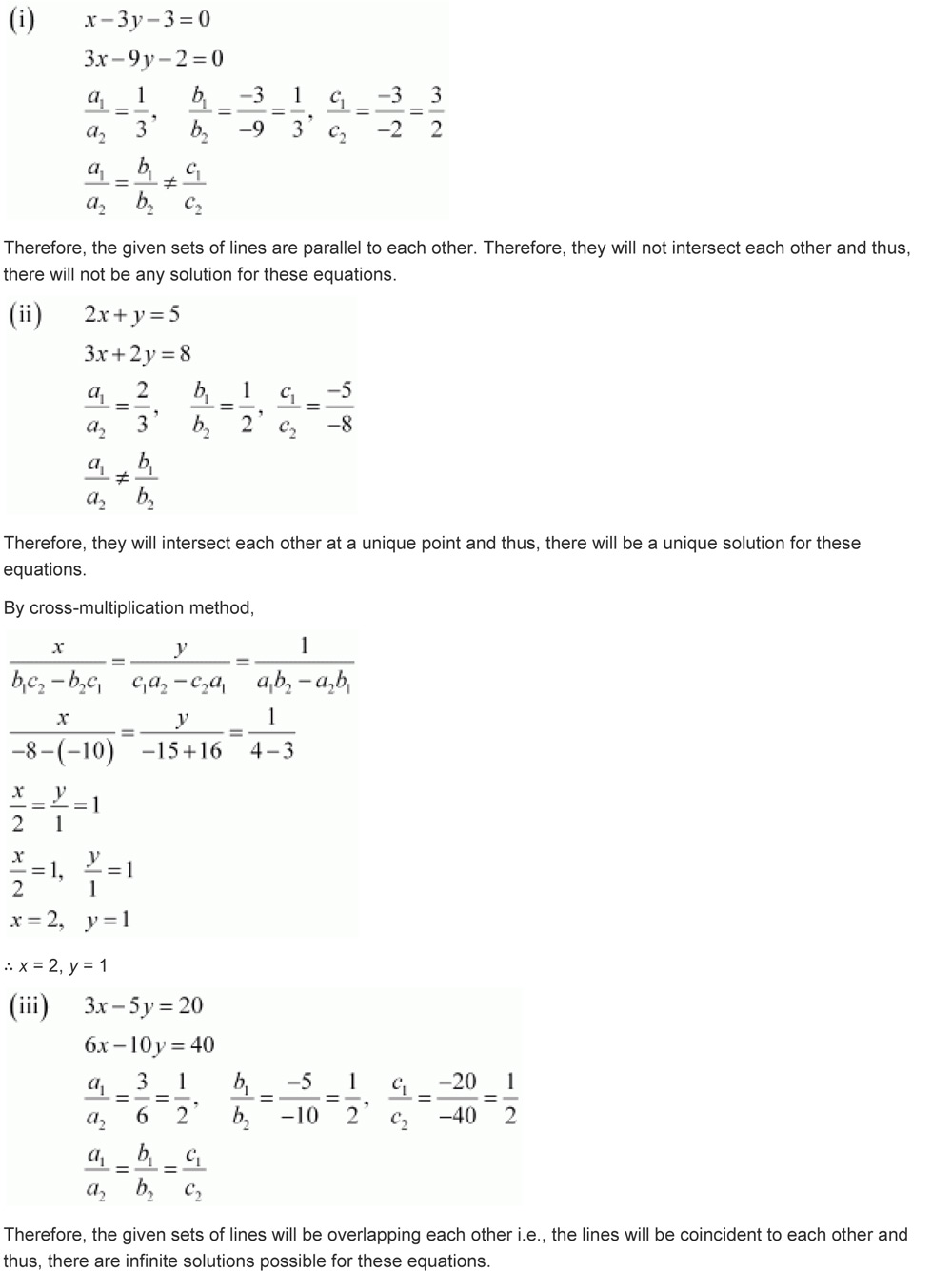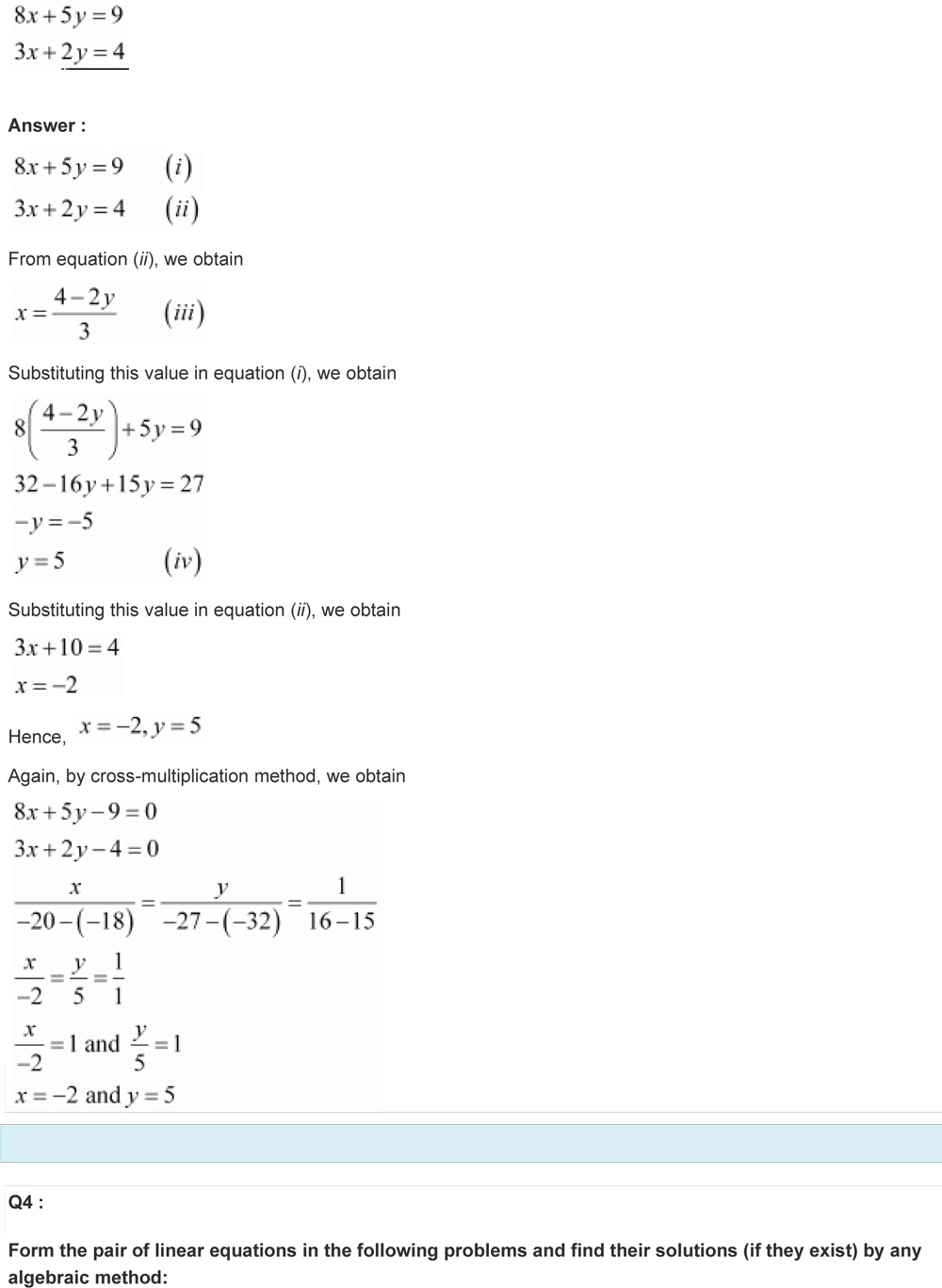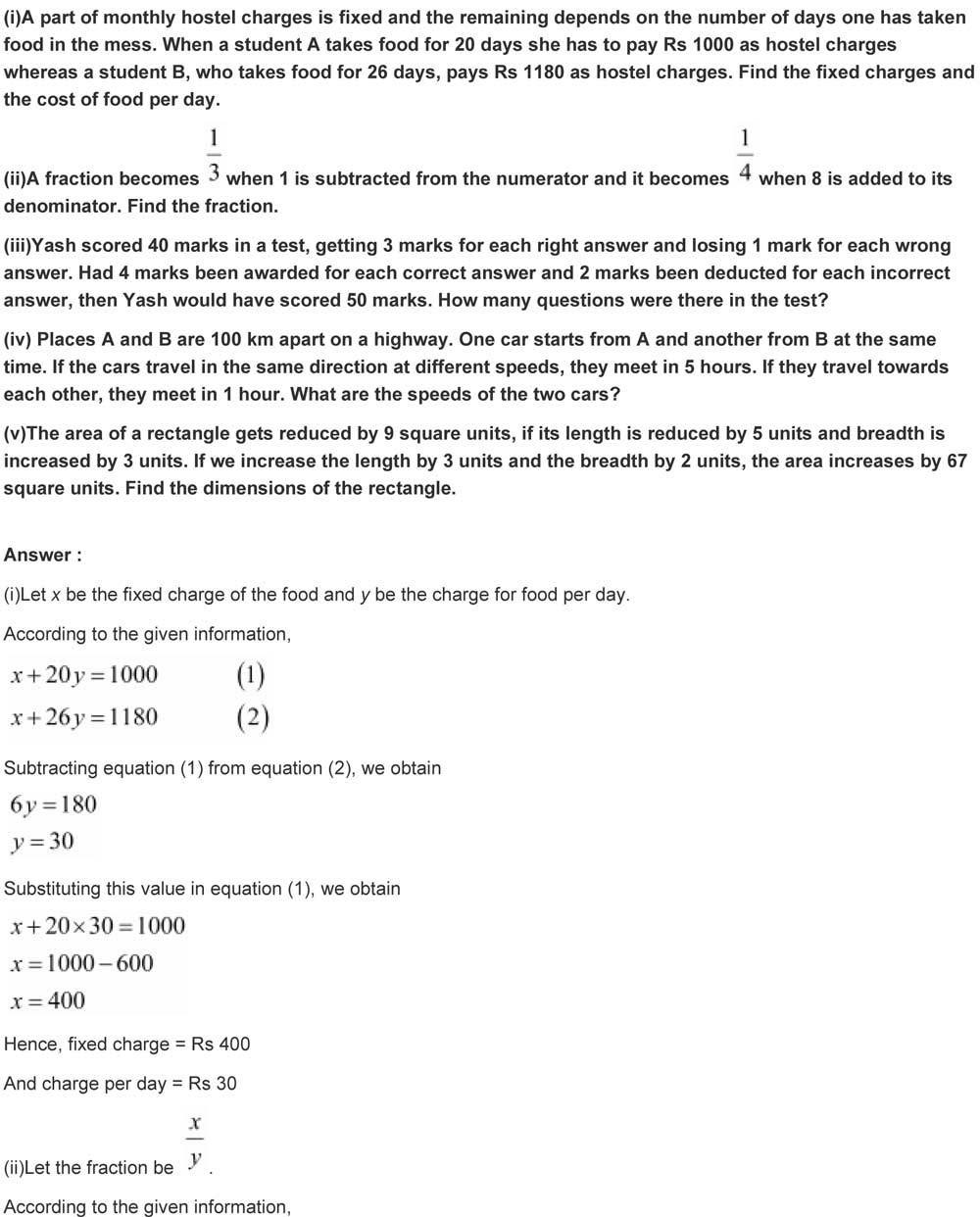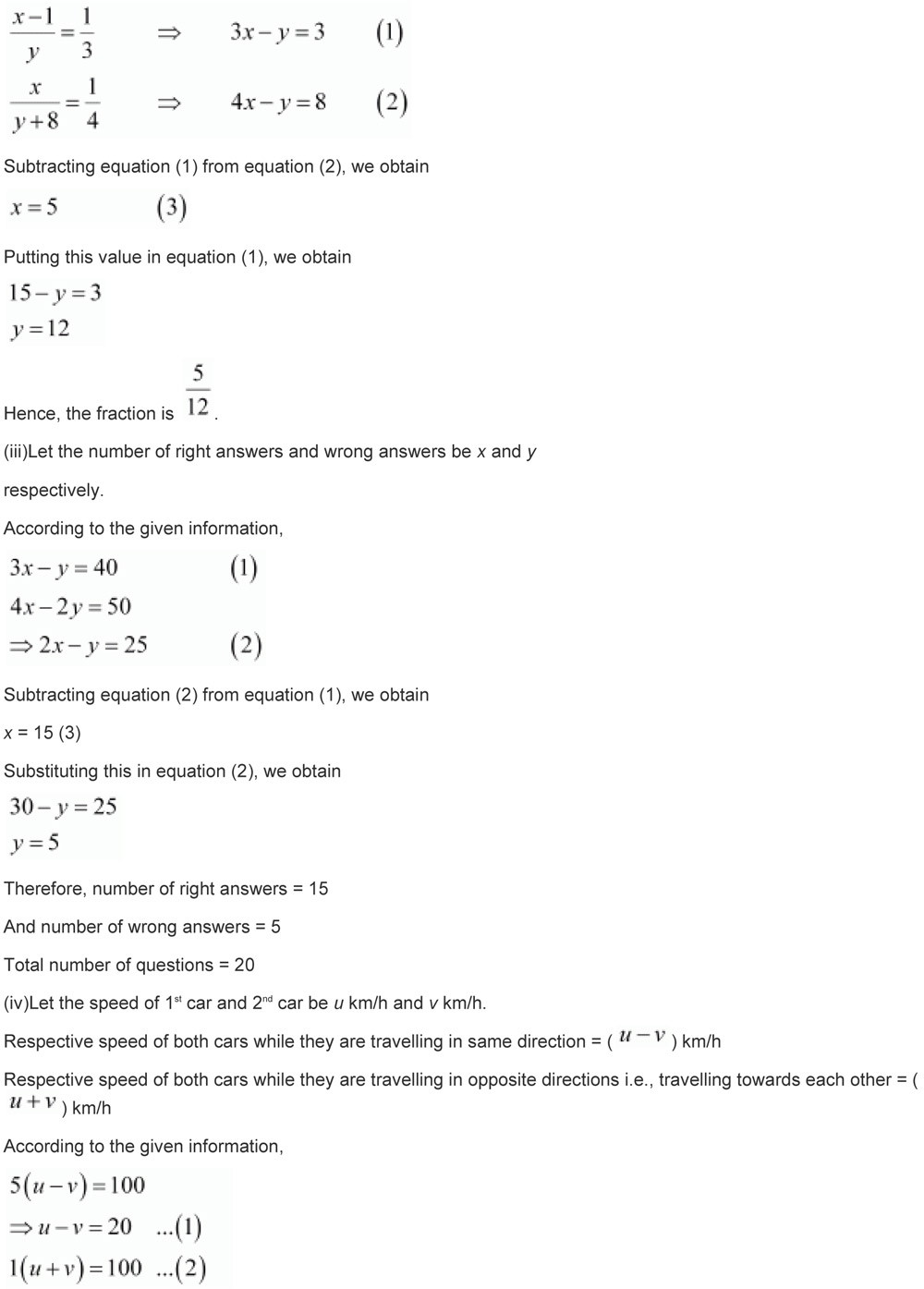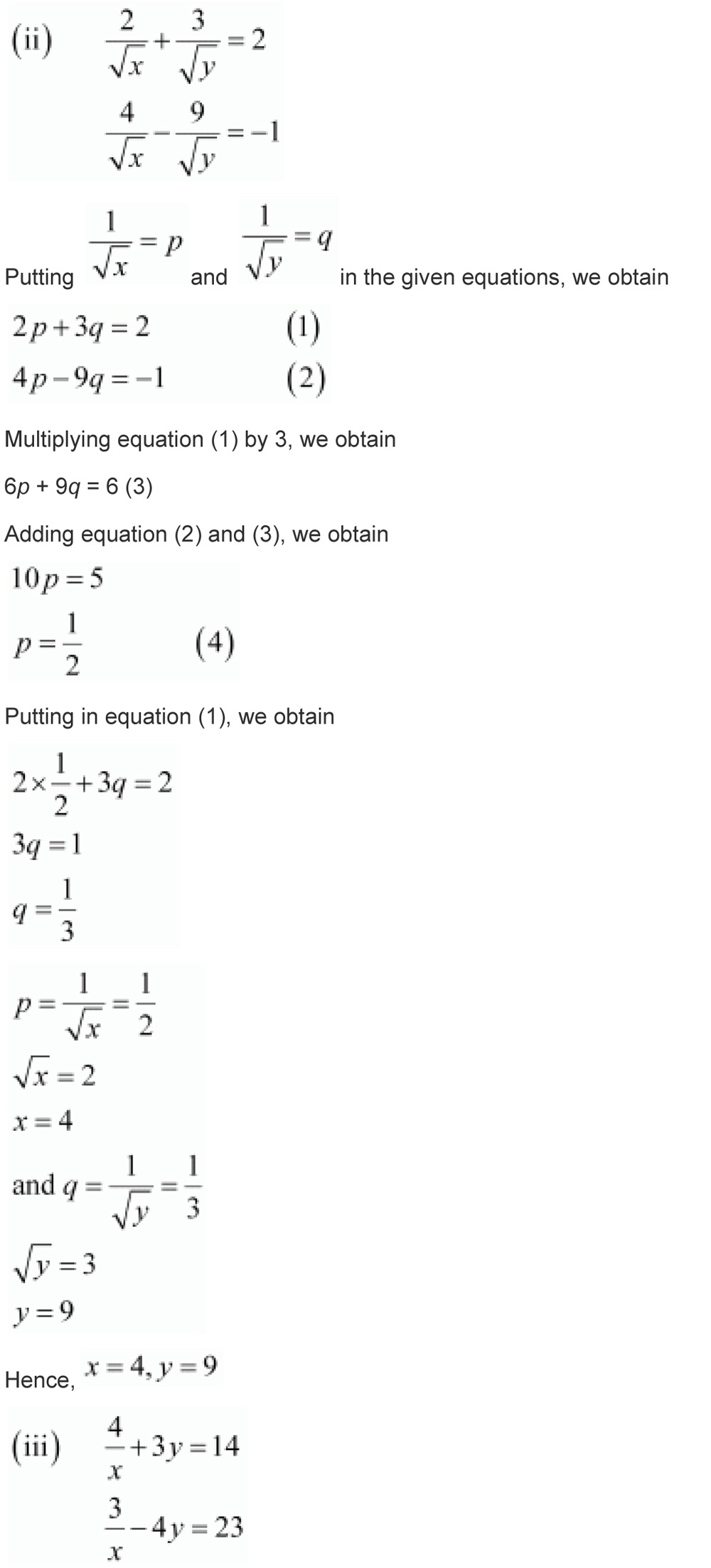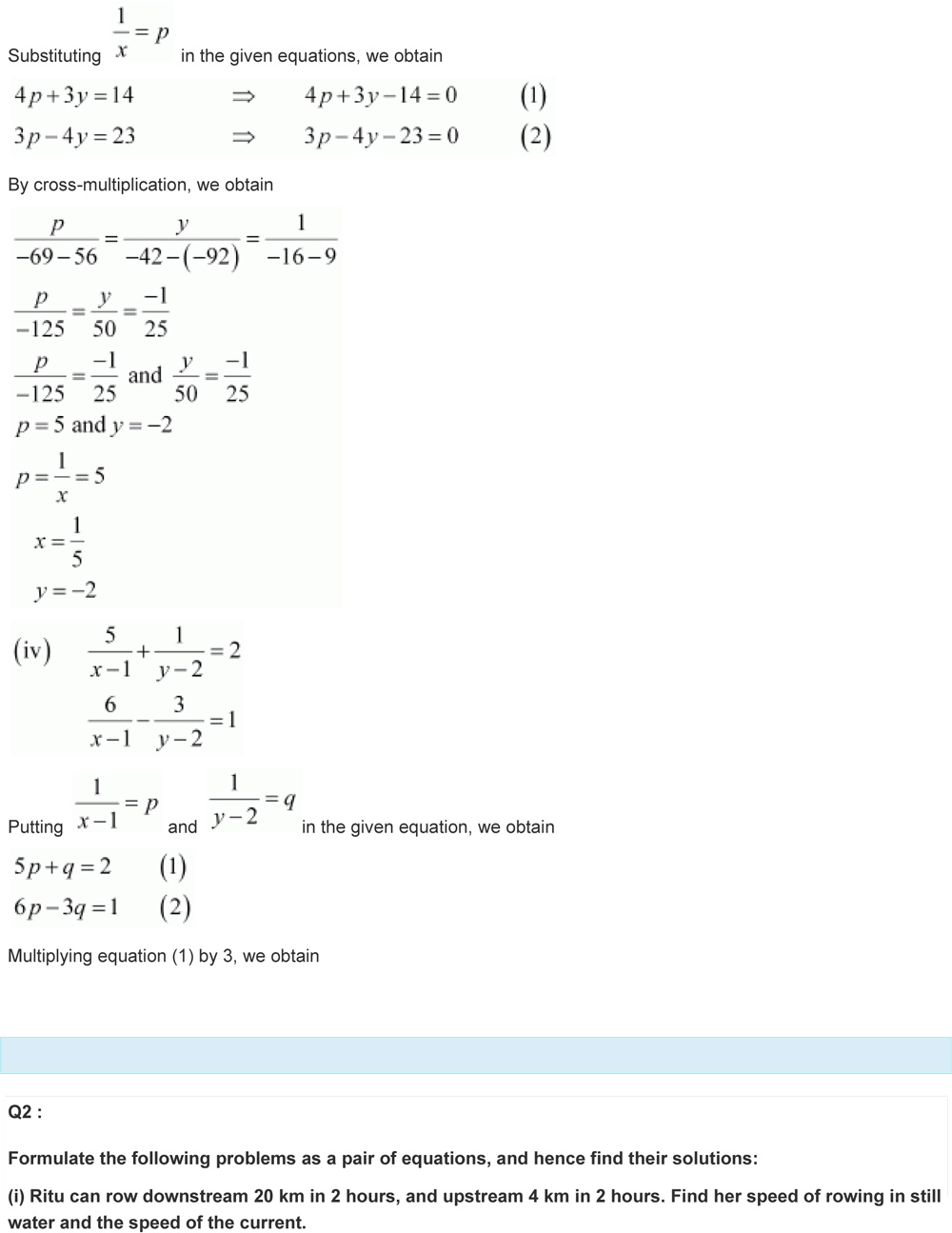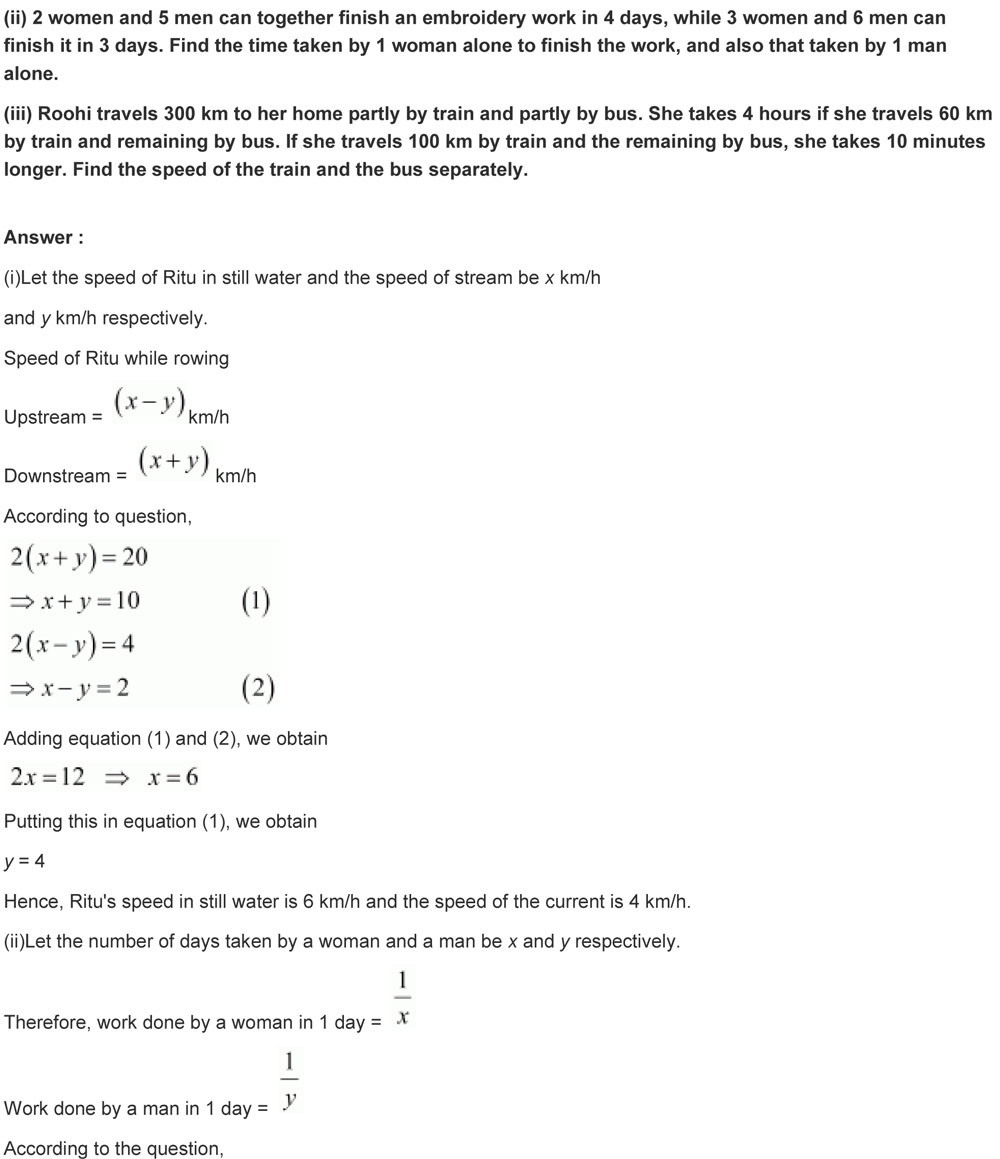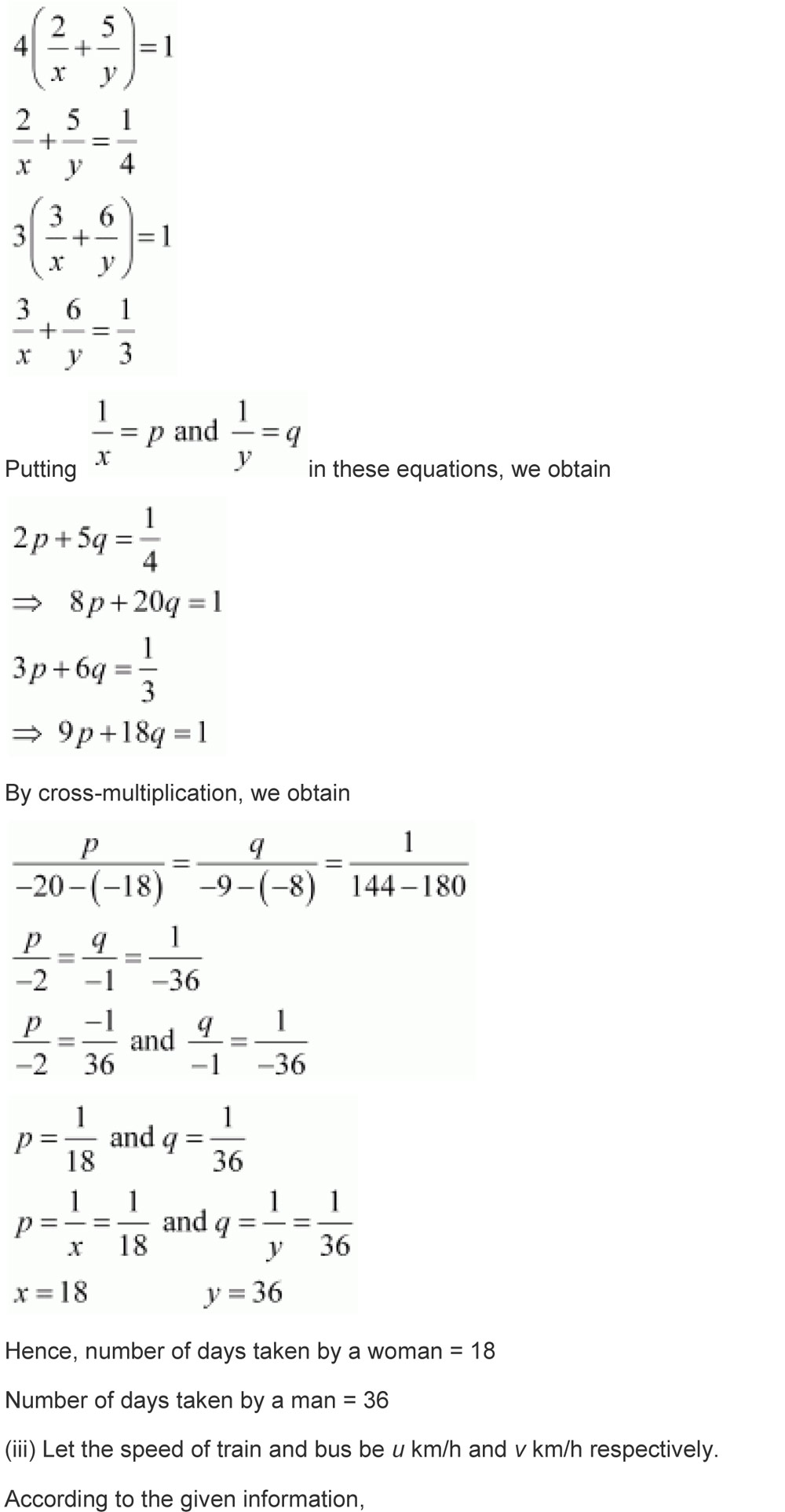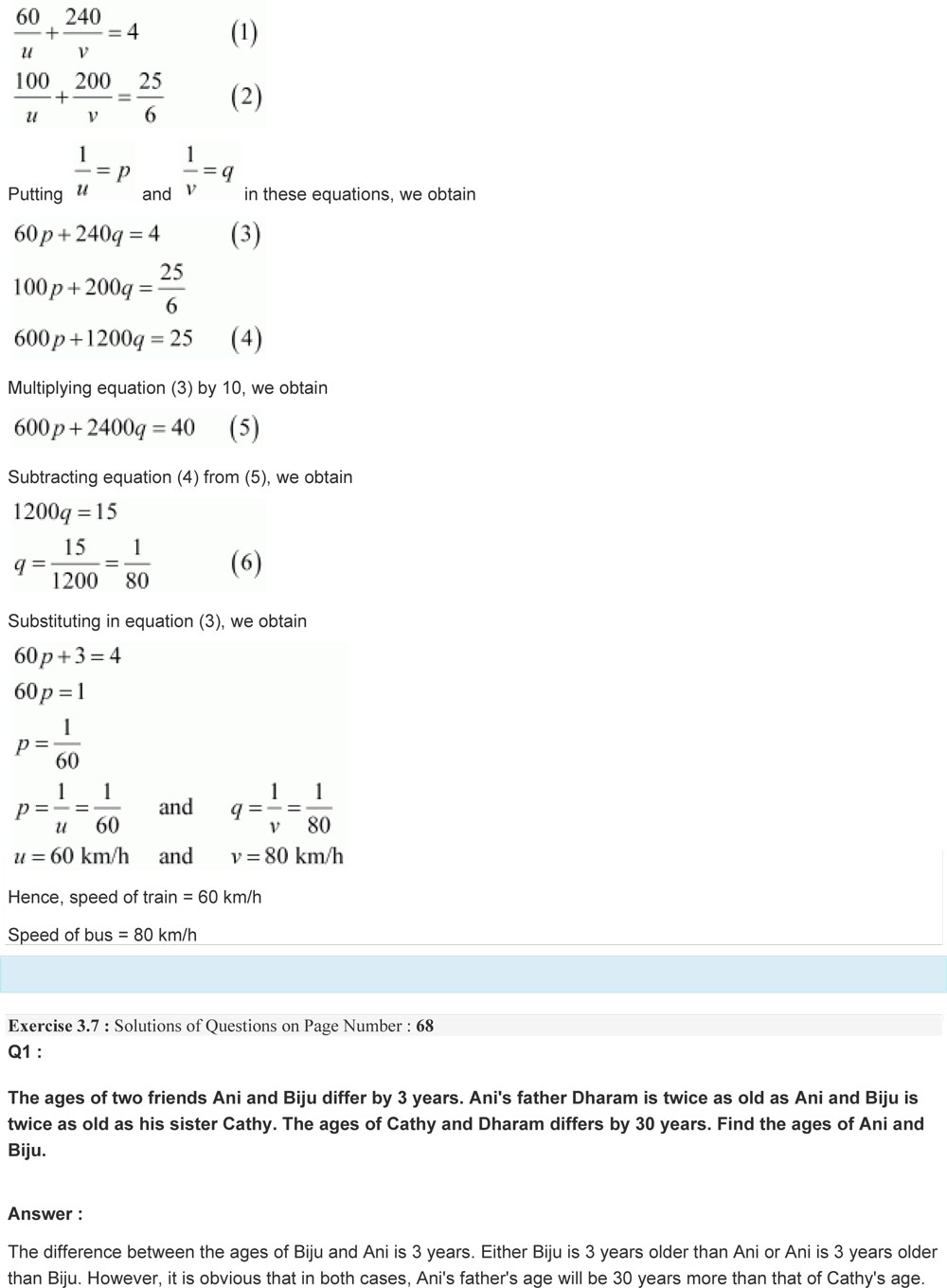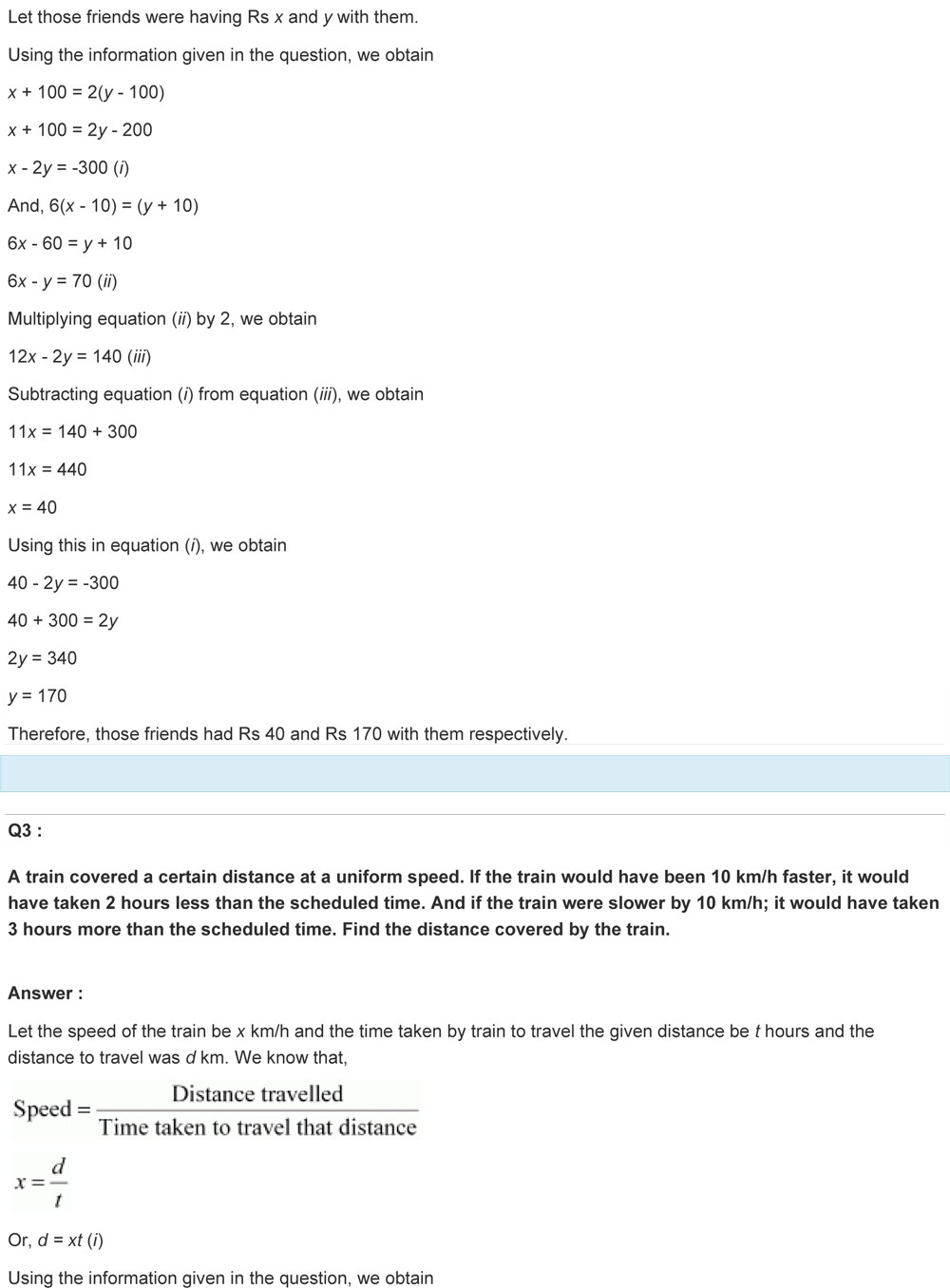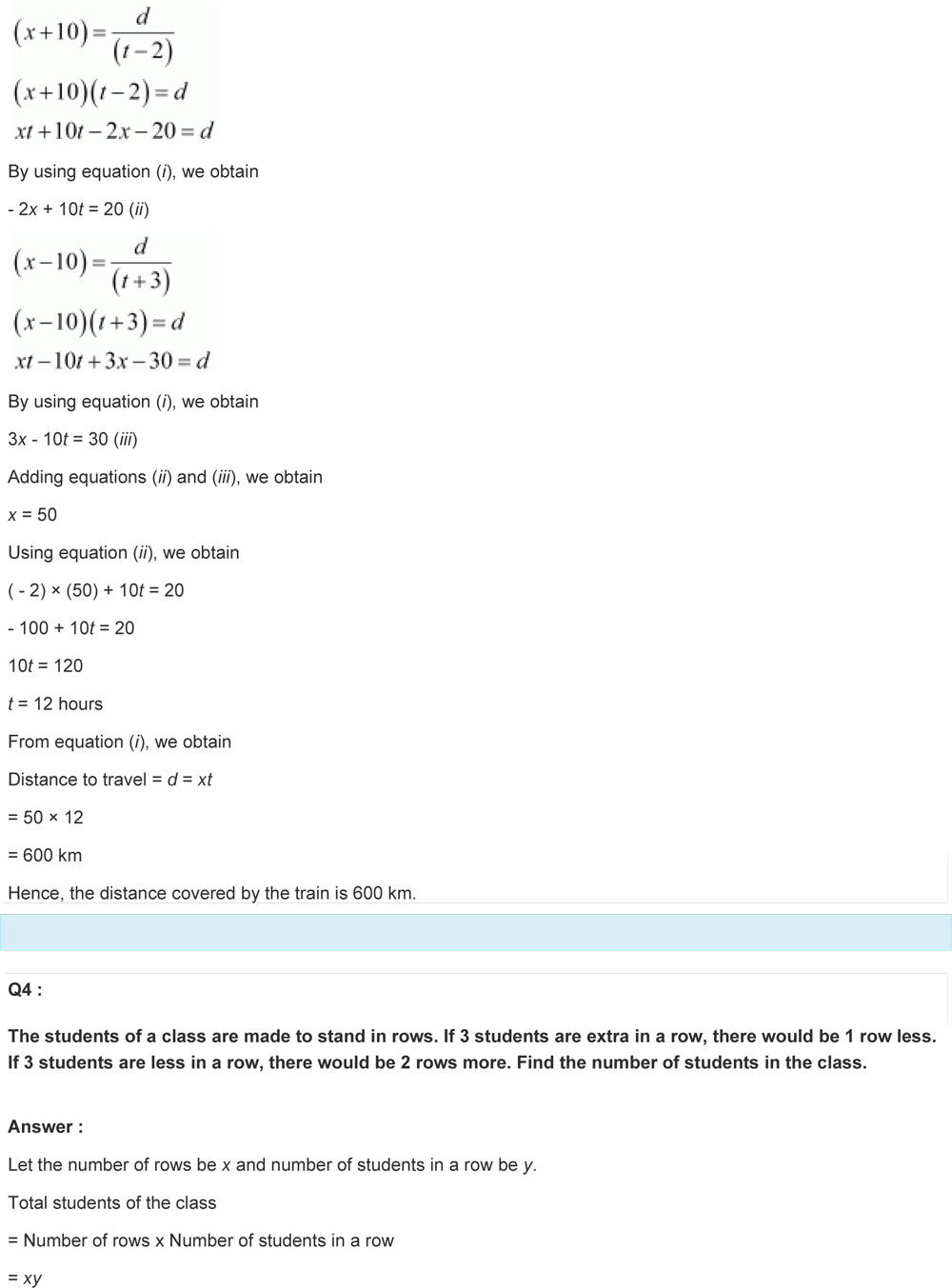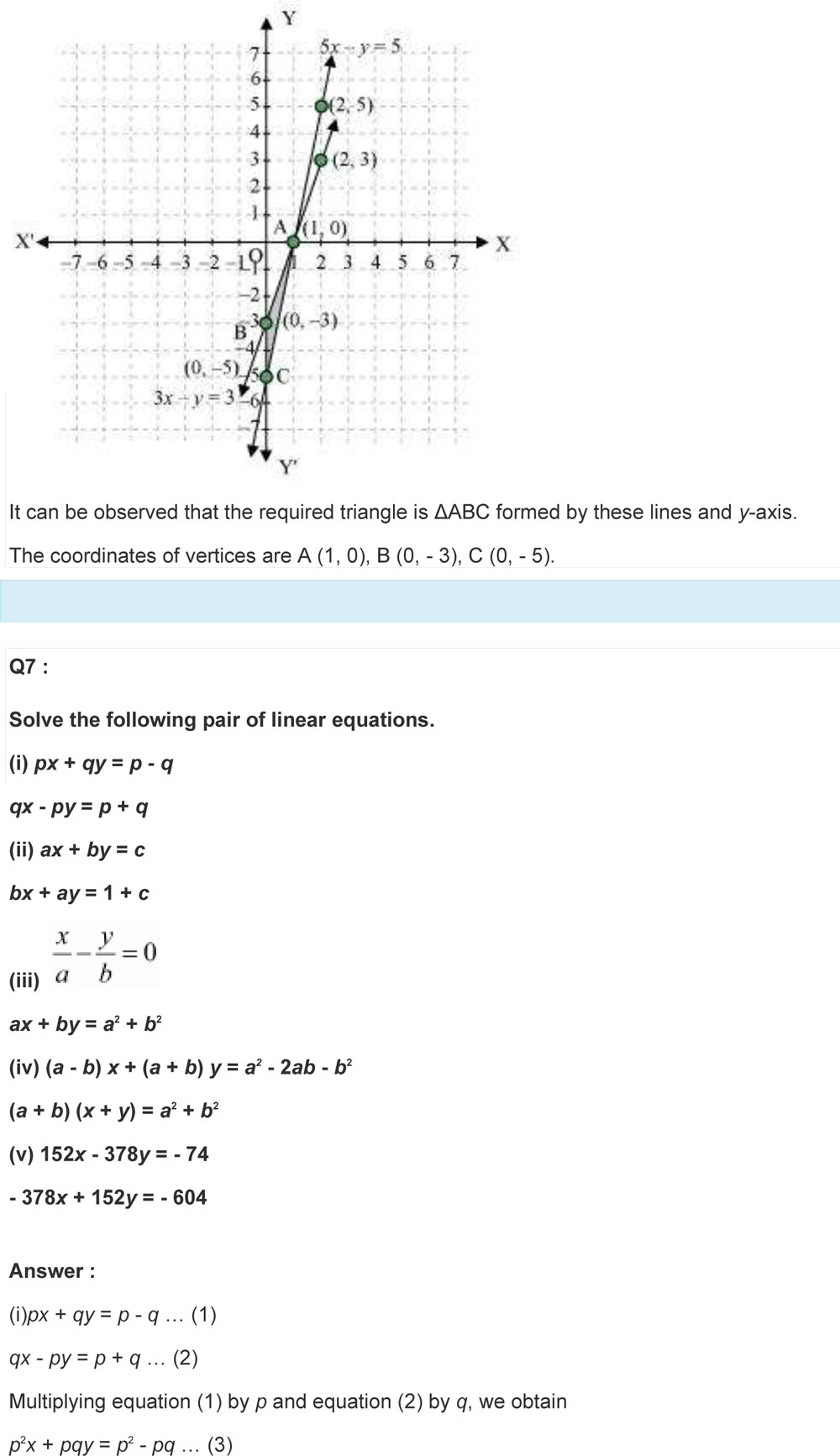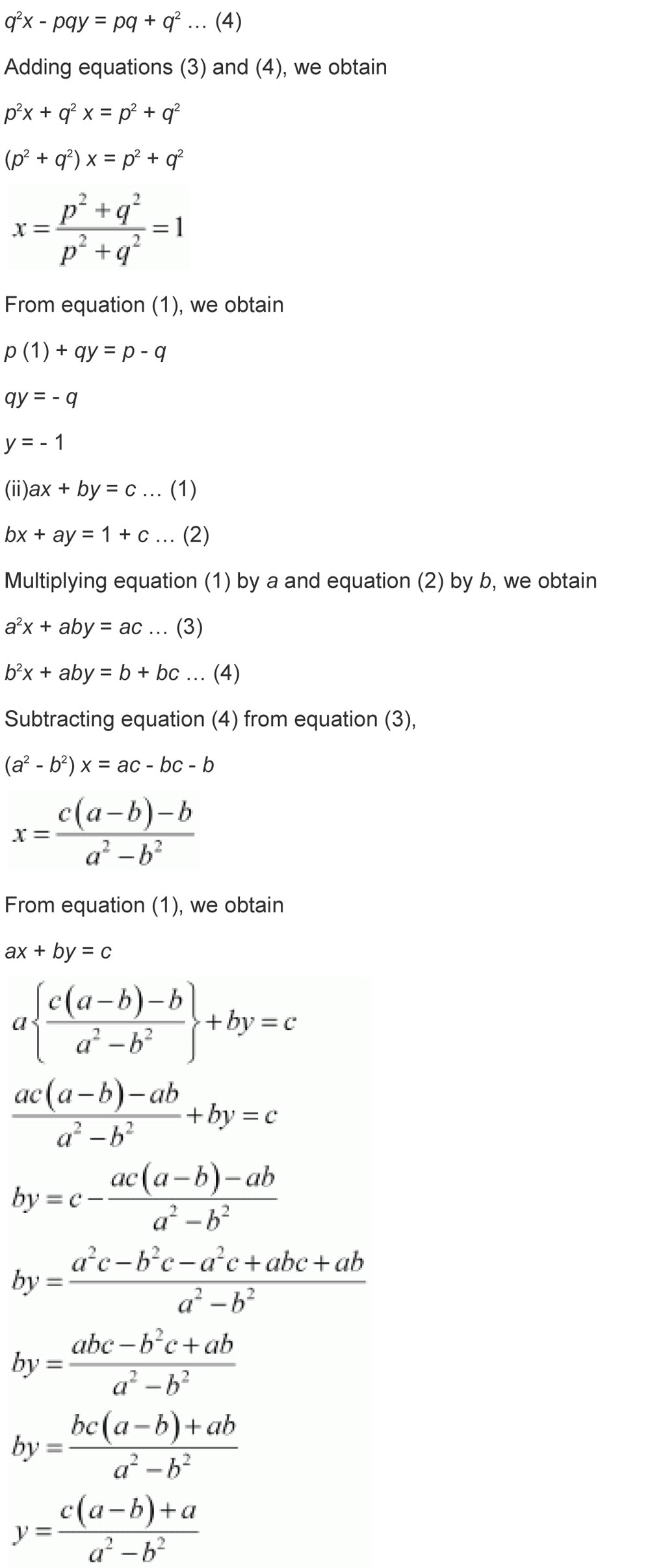### 1 thought on “Linear Equations in Two Variables Class 10 NCERT Solutions”

1. Pingback: Study tips to score well in the CBSE Board Exam – Axioms Education